## Logarithmic Spiral Evolute(1)

Using(2)

gives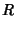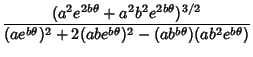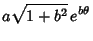(3)

and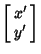(4)

so(5)

and the Tangent Vector is given by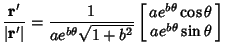(6)

The coordinates of the Evolute are therefore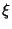(7)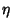(8)

So the Evolute is another logarithmic spiral with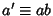, as first shown by Johann Bernoulli.However, in some cases, the Evolute is identical to the original, as can be demonstrated by making the substitution to the new variable(9)

Then the above equations become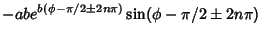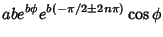(10)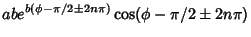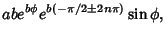(11)

which are equivalent to the form of the original equation if(12)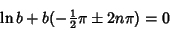(13)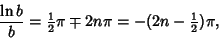(14)

where only solutions with the minus sign in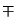exist. Solving gives the values summarized in the following table.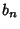1 0.2744106319...2 0.1642700512...3 0.1218322508...4 0.0984064967...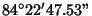5 0.0832810611...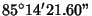6 0.0725974881...7 0.0645958183...8 0.0583494073...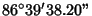9 0.0533203211...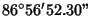10 0.0491732529...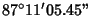References

Lauwerier, H. Fractals: Endlessly Repeated Geometric Figures. Princeton, NJ: Princeton University Press, pp. 60-64, 1991.